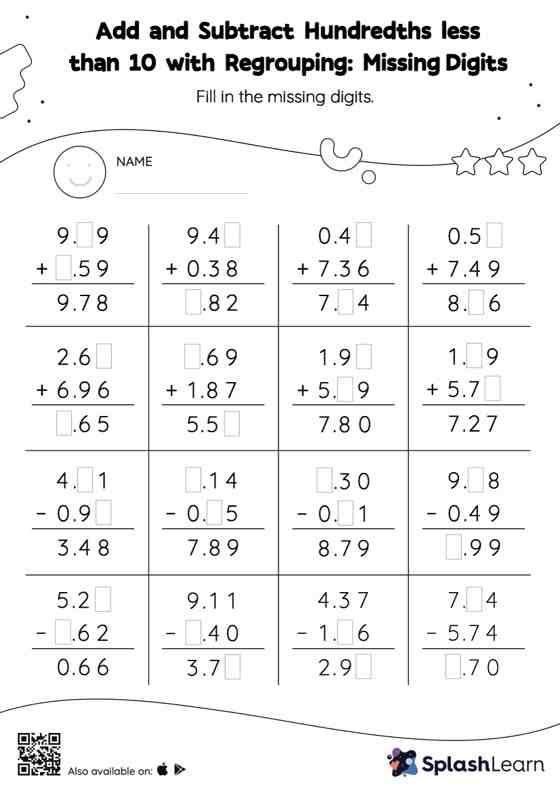# Add and Subtract Hundredths less than 10 with Regrouping: Missing Digits Worksheet

Home > Add and Subtract Hundredths less than 10 with Regrouping: Missing DigitsThis add and subtract hundredths less than 10 with regrouping worksheet consists of a set of questions on addition and subtraction, which help students develop fluency. Students find the missing number while adding or subtracting decimals by using the relationship between addition and subtraction. To reach the answer, they also use the relationship between ones, tenths, hundredths, thousandths, etc., To regroup the numbers in add and subtract hundredths less than 10 with regrouping worksheet. This worksheet is about practicing the column method in which numbers are written one on top of another. In this method, students use their place value understanding to solve the problems.